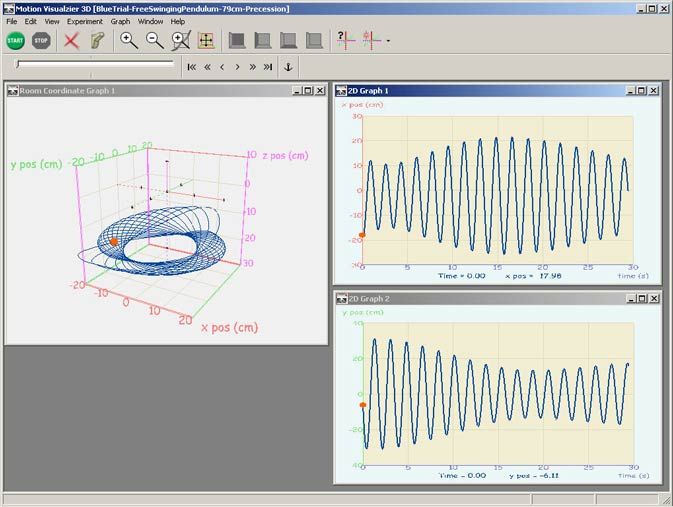The 3D graph shows the motion of a free-swinging pendulum. The graph on the upper right is the x-coordinate (red axis on 3D graph) vs. time, and the lower right graph is the y-coordinate (green axis on 3D graph) vs. time. The two time graphs together show how the greatest excursion of the pendulum moved between the x- and y-coordinates. The pendulum swung in an ellipse that rotated in time. << Back